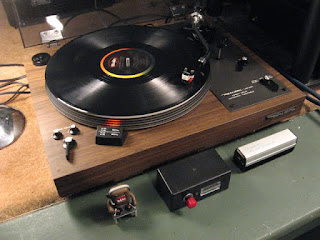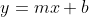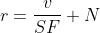## Friday, April 6, 2012

### AVC: Precision Gyro Calibration Reloaded

Here's the updated 2012 version of the gyro calibration I performed on Data Bus in 2011.

The goal as before is improved heading accuracy. Target: L3G4200D on a Pololu minIMU-9.

Last time I used my "high precision rate gyro calibrator," a 1970's Realistic Lab-400 Direct Drive turntable. I used it again this time. I also used by gyro/magnetometer calibration machine.High Precision Gyro Rate CalibratorGyro/Magnetometer calibraiton machine.
Alright then. Let's get on with it...

Part 1: Turntable

The Theory
To calibrate, we need to collect three known rotational speeds (0, 45 rpm or 270°/sec, and 33-1/3 rpm or 200°/sec). Plotting gyro reading versus known rpm traces a line. Then, solve the straight line function that relates gyro reading to heading rate of change in degrees per second. Remember y-intercept form?The second equation is written in terms of converting from known gyro readings and known heading rates.  The scale factor SF where m = 1/SF in °/sec. We divide the gyro raw gyro value v (y-axis) by this. Then adjust by the null point N aka b. N is equivalent to the raw, null (sitting still) gyro reading divided by SF.

Data Collection
The null value was easy; sit the robot still, read gyro data. To get the 33-1/3 and 45 rpm data, I placed the entire 5 lbs robot on the turntable, elevated above the delicate turntable arm by a coffee can. Rest assured, I removed the expensive stylus before testing.

Direct drive turntables use a strobe shining on a pattern on the platter to help the user adjust speed. The strobe is based on the electrical outlet frequency which may vary ever so slightly over time. I created my own strobe by programming my LPC2103 breakout board to blink an LED at 60Hz using a timer peripheral and interrupts. It was a good opportunity to learn about ARM7 timer setup. Here's the source.

My DMM registered the ARM's LED frequency at 59.8Hz, a 0.33% discrepancy I can't explain. The DMM measures the wall outlet frequency at 60.0Hz. The crystal is rated at ±30ppm tolerance and ±50ppm stability.

Data Analysis

This time around I have Octave at my disposal so no wasting time writing Perl scripts to do simple averages. After splitting the data into 3 files with 0,  45 rpm (270°/sec) and 33.33 rpm (200°/sec) data, just do this:

mean(G0(:,4))
ans =  2.5654

mean(G33(:,4))
ans = -2854.5

mean(G45(:,4))
ans = -3857.9

So let's find SF in terms of the raw ADC conversion value. Put together the data for the three points:

X=[0, 200, 270];
Y=[mean(G0(:,4)), mean(G33(:,4)), mean(G45(:,4))];

Calculate slope:

(Y(:,1)-Y(:,2))/(X(:,1)-X(:,2))
ans = -14.285

and

(Y(:,3)-Y(:,2))/(X(:,3)-X(:,2))
ans = -14.334

I guess we can just average those results: SF = -14.309  Also, N = - 0.1793. When it comes time to convert to °/sec, calculate (interpolate) using the bias (null) and SF in raw ADC value:

float gyroRate(unsigned int adc)
{
return ((float) adc - 2.5654) / -14.309;
}

Or you can do return ((float) adc)/-14.309 + 0.1793;

Part 2: Gyro Machine

The Theory
Let's try the calibration again, using my gyro/magnetometer machine. It's a turntable with built in encoder with 2 degree resolution. The encoder sensors interface with Data Bus so the robot has access to reference heading data.

The idea is to spin the robot and turntable around a few times at varying rates while recording the machine's reference heading and the reported gyro reading.

Then, using a solver in Octave, find the offset and scale (N and SF) that results in a best fit of the gyro data to the machine reference data.

Data Collection
I wrote a simple function to record time, gyro data, and total heading from the gyro machine. This function obtains data from the Sensor object which is updated at 50Hz. The data is written out to a logfile which I can send to the PC from the onboard shell using my serial terminal program.

Data Analysis
An Octave script I put together makes use of the sqp()solver. It's simpler than it sounds.

We're interested in a two-variable problem: scale and offset. Set up an X0 vector populated with an initial guess at scale and offset. It could be [1 0]. Or we could use the values we calculated up above [-14.309 0.1793].

Now, write the function to calculate the sum of squared error, call it phi(). We're going to use the heading rate, rather than heading as that ended up providing a better fit. The gyro provides heading rate, of course, but the machine provides heading.

Convert to heading rate via gradient(H, T/1000) where H is the reported total heading and T is the time in milliseconds which we divide by 1000 to scale to seconds.

Calculate heading rate from the raw gyro data with G/X(1)-X(2)) where X(1) is scale and X(2) is offset. Now we put it all together, finding the error, and the sum of squared error.

% solver function to calculate SSE
%
% X -- vector, [scale, offset]
function sse=phi(X)
global D;
%heading consisting of reference and integration of gyro
%ERR = (GH - D(:,2));
sse = sumsq(ERR);
end
sse = sumsq(ERR);
end

That's the hard part. And it wasn't even all that hard. The main routine sets the initial guess and calls sqp(), passing X0 and phi.

X0 = [ -14, 0 ];
[X, obj, info, iter, nf, lambda] = sqp(X0, @phi)

The function I wrote takes three parameters, Time, Gyro, and Heading, populates a global data matrix D, then runs the two lines above, then plots the gyro data adjusted by scale and offset, and returns the optimized values for scale and offset. Here's what we get.

The fit is within about 3 degrees on the heading. The optimized scale and offset are:

scale =  -14.49787
offset =  -0.20168

Interestingly, scale is very close (1.30% difference) to the result from the turntable. That makes me feel a bit better about both approaches. I need to understand how and why the offset varies.

Temperature

Temperature will affect null (zero point).The datasheet claims the value is at most 0.04dps/°C which is about 144 degrees per hour, which I consider significant enough to worry about. I'll need to calibrate at different temperature points and attempt to plot offset and scale factor versus temperature.